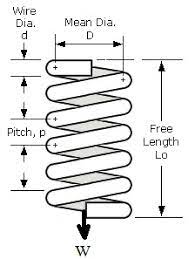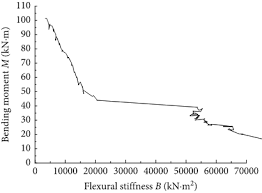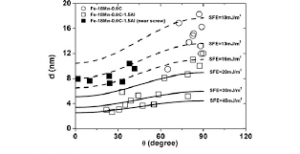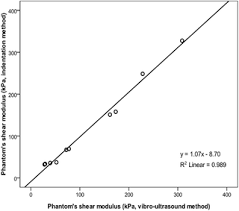## How to Calculate and Solve for Deflection of Coil of Spring | Material SelectionThe image above represents deflection of coil of spring.

To compute for deflection of coil of spring, four essential parameters are needed and these parameters are Force (F), Centre to Centre Diameter of Spring (D), Cross-section Diameter of Spring (d) and Shear Modulus (G).

The formula for calculating deflection of coil of spring:

σc = 8FD³/d4G

Where:

σc = Deflection of Coil of Spring (G)
F = Force
D = Centre to Centre Diameter of Spring
d = Cross-section Diameter of Spring
G = Shear Modulus

Let’s solve an example;
Find the deflection of coil of spring when the force is 8, the centre to centre diameter of spring is 2, the cross-section diameter of spring is 4 and the shear modulus is 6.

This implies that;

F = Force = 8
D = Centre to Centre Diameter of Spring = 2
d = Cross-section Diameter of Spring = 4
G = Shear Modulus = 6

σc = 8FD³/d4G
σc = 8(8)(2)³/(4)4(6)
σc = 8(8)(8)/(256)(6)
σc = 512/1536
σc = 0.33

Therefore, the deflection of coil of spring is 0.33 m.

## How to Calculate and Solve for Stiffness Performance Index | Material SelectionThe image above represents stiffness performance index.

To compute for stiffness performance index, two essential parameters are needed and these parameters are Shear Modulus (G) and Density (ρ).

The formula for calculating stiffness performance index:

P = √(G)/ρ

Where:

P = Stiffness Performance Index
G = Shear Modulus
ρ = Density

Let’s solve an example;
Find the stiffness performance index when the shear modulus is 36 and the density is 12.

This implies that;

G = Shear Modulus = 36
ρ = Density = 12

P = √(G)/ρ
P = √(36)/12
P = 6/12
P = 0.5

Therefore, the stiffness performance index is 0.5

Calculating the Shear Modulus when the Stiffness Performance Index and the Density is Given.

G = (P x ρ)2

Where:

G = Shear Modulus
P = Stiffness Performance Index
G = Shear Modulus= Stiffness Performance Index
ρ = Density

Let’s solve an example;
Find the shear modulus when the stiffness performance index is 25 and the density is 5.

This implies that;

P = Stiffness Performance Index = 25
ρ = Density = 5

G = (P x ρ)2
G = (25 x 5)2
G = 15,625

Therefore, the shear modulus is 15,625.

## How to Calculate and Solve for Partial Dislocation Separation | Fracture MechanicsThe image above represents partial dislocation separation.

To compute for partial dislocation separation, four essential parameters are needed and these parameters are Shear Modulus (G), Burger Vector (b2), Burger Vector (b3and Stacking Fault Energy (γ).

The formula for calculating partial dislocation separation:

d = Gb2b3 / 2πγ

Where:

d = Partial Dislocation Separation
G = Shear Modulus
b2 and b3 = Burger Vectors
γ = Stacking Fault Energy

Let’s solve an example;
Find the partial dislocation separation when the shear modulus is 4. the burger vectors is 8 and 10, the stacking fault energy is 14.

This implies that;

G = Shear Modulus = 4
b2 and b3 = Burger Vectors = 8 and 10
γ = Stacking Fault Energy = 14

d = Gb2b3 / 2πγ
d = (4)(8)(10) / 2π(14)
d = 320 / 87.96
d = 3.64

Therefore, the partial dislocation separation is 3.64 m.

## How to Calculate and Solve for Shear Modulus | Rock MechanicsThe image above represents shear modulus.

To compute for shear modulus, two essential parameters are needed and these parameters are young’s modulus (E) and Poisson’s ratio (v).

The formula for calculating the shear modulus:

G = E / 2(1 + v)

Where:

G = Shear Modulus
E = Young’s Modulus
v = Poisson’s Ratio

Let’s solve an example;
Find the shear modulus when the young’s modulus is 32 and the Poisson’s ratio is 24.

This implies that;

E = Young’s Modulus = 32
v = Poisson’s Ratio = 24

G = E / 2(1 + v)
G = 32 / 2(1 + 24)
G = 32 / 2(25)
G = 32 / 50
G = 0.64

Therefore, the shear modulus is 0.64.

Calculating the Young’s Modulus when the Shear Modulus and the Poisson’s Ratio is Given.

E = G (2 + 2v)

Where:

E = Young’s Modulus
G = Shear Modulus
v = Poisson’s Ratio

Let’s solve an example;
Find the young’s modulus when the shear modulus is 12 and the Poisson’s ratio is 10.

This implies that;

G = Shear Modulus = 12
v = Poisson’s Ratio = 10

E = G (2 +2v)
E = 12 (2 + 2(10))
E = 12 (2 + 20)
E = 12 (22)
E = 264

Therefore, the young’s modulus is 264.